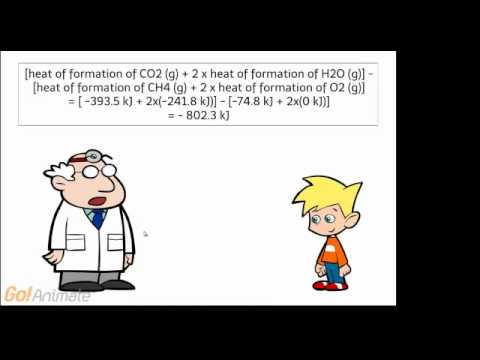### Main Difference

Thermodynamics is the branch of physics that deals with the study of heat and other relating phenomena. This also deals with the relations of heat with other forms of energy such as electrical, mechanical or chemical energy. Entropy and Enthalpy are the famous terms related to thermodynamics. Entropy is the measurement of the disorder or the randomness in the system during the chemical process, whereas enthalpy measures the heat change or internal energy change of a system during the chemical reaction under constant pressure. Actually, enthalpy is the measure of total energy in the system, although it always denotes the change in the energy system at constant pressure as it as the total enthalpy of the system can’t be measured. It is measured in joules per kilogram. On the other hand, entropy is the measure of random activity, which is usually the amount of disorder in the system. The SI unit for Entropy (S) is Joules per Kelvin (J/K).

### Comparison Chart

 Entropy Enthalpy Definition Entropy is the measurement of the disorder or the randomness in the system during the chemical process. Enthalpy measures the heat change or internal energy change of a system during the chemical reaction under constant pressure. SI unit Unit joule per kelvin (J/K) The SI unit for specific enthalpy is joule per kilogram. Formula S=q/T H=U+PV

What is Entropy?

Entropy is the measurement of the disorder or the randomness in the system during the chemical process. Actually, it is the measure of random activity, which is usually the amount of disorder in the system. As we know that energy in a body tells about its capability to do work, the energy in a body can be of any type. It can be mechanical, chemical, thermal, nuclear or any other energy. The measure of that change of energy or disorder during the chemical process is entropy. ‘S’ denotes entropy, and it is always written as a capital letter. In an equation, it is written as ‘ΔS’ as it represents the disorder in the entropy during the chemical process. The SI unit for Entropy (S) is Joules per Kelvin (J/K). The temperature in the entropy equation is measured on the absolute or Kelvin temperature scale.

What is Enthalpy?

Enthalpy measures the heat change or internal energy change of a system during the chemical reaction under constant pressure. Enthalpy is the measure of total energy in the system, although it always denotes the change in the energy system at constant pressure as it as the total enthalpy of the system can’t be measured. Enthalpy is denoted as ‘ΔH,.’ It tells about the difference between the enthalpy of the products and the enthalpy of the reactants. It is measured in the units of Jmol-1. The word enthalpy is derived from the Greek word ‘enthalpos,’ meaning ‘to put heat into’. The word ‘enthalpy’ for this measure was coined by a 19th-century famous physicist Heike Kamerlingh Onnes. It is generally measured in joules per kilogram.

Entropy vs. Enthalpy

• Entropy is the measurement of the disorder or the randomness in the system during the chemical process, whereas enthalpy measures the heat change or internal energy change of a system during the chemical reaction under constant pressure.
• Actually, enthalpy is the measure of total energy in the system, whereas entropy is the measure of random activity, which is usually the amount of disorder in the system.
• The word ‘enthalpy’ for this measure was coined by a 19thcentury famous physicist Heike Kamerlingh Onnes, on the other hand, German physicist Rudolf Clausius coined the word ‘entropy.’

Explanatory Video